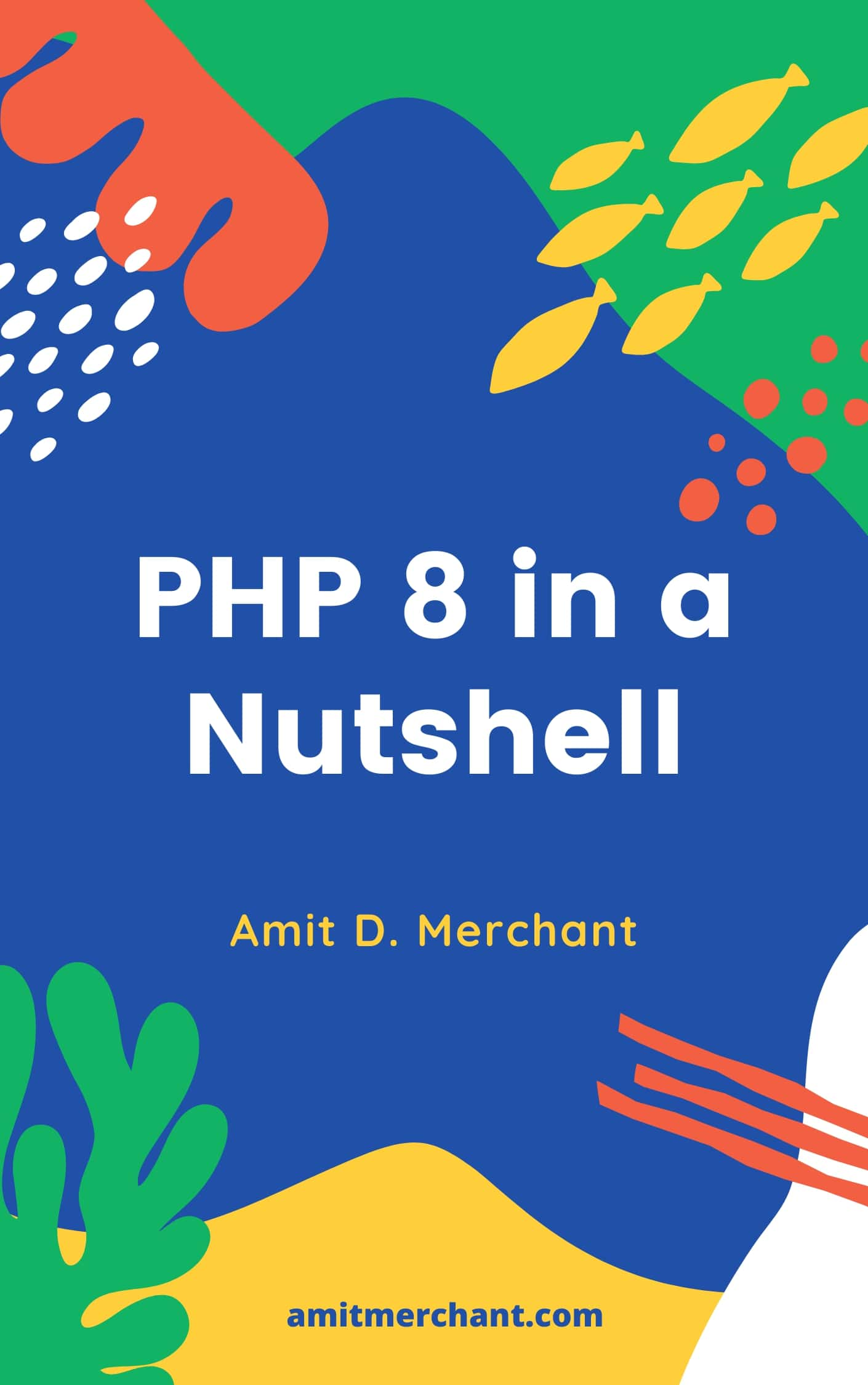# Amit MerchantVerified (\$10/year for the domain)

A blog on PHP, JavaScript, and more

# Convert any value to a boolean in JavaScript

November 29, 2022 ·

When you’re working with JavaScript, you often stumble upon a situation where you need to check certain conditions. And often, you would need a boolean value (`true` or `false`) or boolean expression to check for the condition.

Many times, you would deduce the boolean value from, a condition, let’s say. But what if you have a value that is not a boolean value and you need to convert it to a boolean value? For instance, you may want to safely convert a string or a number to a boolean value.

In JavaScript, there are a couple of ways to convert any value to a boolean value. Let’s see them one by one.

## The Boolean() object

The first way to convert any value to a boolean value is by using the built-in `Boolean()` object. This function takes a value as an argument and returns a boolean value.

For instance, if you pass a string to the `Boolean()` object, it will return `true` if the string is not empty and `false` if the string is empty.

``````let strBoolValue = Boolean('foo'); // true
let strBoolValue = Boolean(''); // false
``````

Similarly, if you pass a number to the `Boolean()` object, it will return `true` if the number is not zero and `false` if the number is zero.

``````let numberBoolValue = Boolean(1); // true
let numberBoolValue = Boolean(0); // false
``````

If you pass an object/array to the `Boolean()` object, it will return `true` irrespective of whether the object/array is empty or not.

``````let objBoolValue = Boolean({}); // true

let objBoolValue = Boolean({ foo: 'bar' }); // true

let arrBoolValue = Boolean([]); // true

let arrBoolValue = Boolean([1, 2, 3]); // true
``````

If you pass a boolean value to the `Boolean()` object, it will return the same boolean value.

``````let boolBoolValue = Boolean(true); // true
``````

If you pass `null` or `undefined` to the `Boolean()` object, it will return `false`.

``````let nullBoolValue = Boolean(null); // false
let undefinedBoolValue = Boolean(undefined); // false
``````

## The Bang Bang (`!!`) operator

There’s another way to convert any value to a boolean value. And that is by using the Bang Bang (`!!`) operator. This operator is a shorthand and a more convenient way to infer a boolean value from a value.

It works the same way as the `Boolean()` object under the hood. So, all the previous examples will work the same way with the `!!` operator.

``````let strBoolValue = !!'foo'; // true
let strBoolValue = !!''; // false

let numberBoolValue = !!1; // true
let numberBoolValue = !!0; // false

let objBoolValue = !!{}; // true
let objBoolValue = !!{ foo: 'bar' }; // true

let arrBoolValue = !![]; // true
let arrBoolValue = !![1, 2, 3]; // true

let boolBoolValue = !!true; // true

let nullBoolValue = !!null; // false
let undefinedBoolValue = !!undefined; // false
``````

## Converting “true”/“false” to boolean

This is a bonus use case and may not be in the scope of this article. But, I thought it would be a good idea to mention it here.

So, one problem with the above methods is that they don’t convert the string `"true"` or `"false"` to their respective boolean values.

For instance, if you pass the string `"false"` to the `Boolean()` function, it will still return `true` instead of `false`.

To get around this, you can use the `JSON.parse()` function to convert the string to a boolean value like so.

``````let yesBoolValue = JSON.parse('true'); // true

let noBoolValue = JSON.parse('false'); // false
``````

Just make sure you don’t pass any other values or empty strings to the `JSON.parse()` function as it will throw an error.

👋 Hi there! I'm Amit. I write articles about all things web development. If you like what I write and want me to continue doing the same, I would like you buy me some coffees. I'd highly appreciate that. Cheers!My Tiny YouTube Channel ⟶